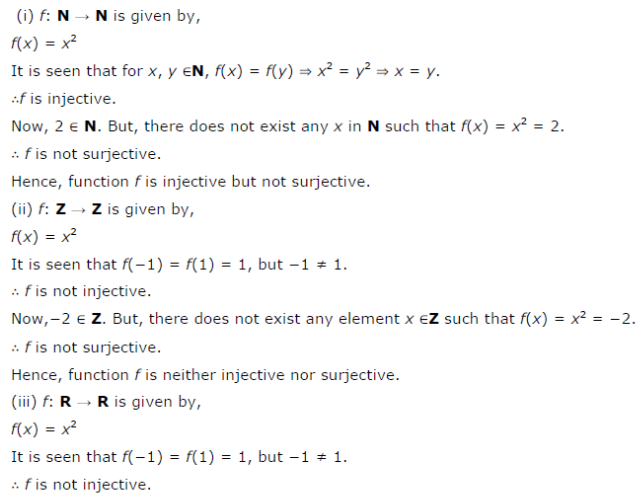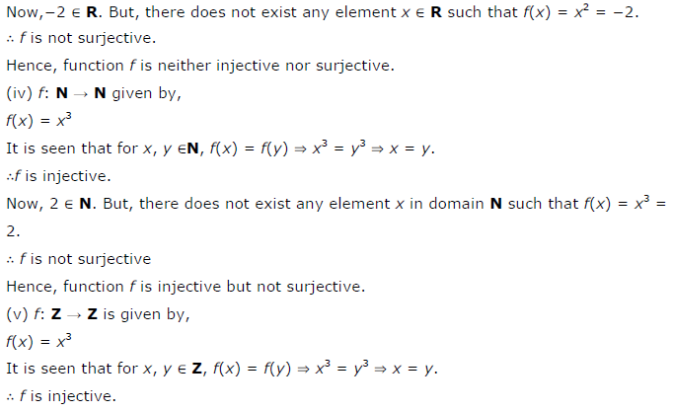# Check the injectivity and surjectivity of the following functions: (i) *f* : **N → N** given by *f(x* ) = x2

Check the injectivity and surjectivity of the following functions:
(i) f : N → N given by f(x ) = x2
(ii) f : Z → Z given by f(x) = x2
(iii) f : R → R given by f(x) = x2
(iv) f : N → N given by f(x) = x3
(v) f : Z → Z given by f(x) = x3